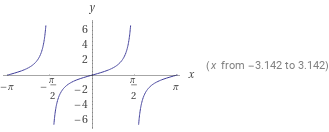Search IntMath
Close

450+ Math Lessons written by Math Professors and Teachers

5 Million+ Students Helped Each Year

1200+ Articles Written by Math Educators and Enthusiasts

Simplifying and Teaching Math for Over 23 Years

# Exploring Tangent in Geometry## What is a Tangent?

A tangent in geometry is a line that touches a circle at one point. That point is called the point of tangency. It is also known as a tangent line. A tangent line is always perpendicular to the radius of the circle at the point of tangency.

The slope of a tangent line is equal to the reciprocal of the radius of the circle. This means that if the radius of the circle is 3, the slope of the tangent line would be 1/3. If the radius is 4, the slope of the tangent line would be 1/4.

## Tangent and Right Angles

The tangent line is always perpendicular to the radius of the circle at the point of tangency. This means that a right angle forms between the tangent line and the radius of the circle. This is known as the tangent-radius theorem.

A right angle is a 90-degree angle. It is formed when two lines meet at a common point, forming a square corner. Right angles are often found in geometry, and they are important for understanding the relationships between different shapes.

## Using Tangent to Measure Circles

Tangent can be used to measure circles. To measure a circle, you first need to find the point of tangency. Then, you can use the slope of the tangent line to determine the radius of the circle.

This process can be used to measure any circle, whether it is a spherical circle or a flat circle. It is also useful for calculating the circumference of a circle.

## Practice Problems

1. Find the slope of a tangent line to a circle with a radius of 5.
Answer: The slope of the tangent line is 1/5.

2. Find the tangent line of a circle with a radius of 6.
Answer: The tangent line is perpendicular to the radius at the point of tangency.

3. Find the angle formed between a tangent line and the radius of a circle with a radius of 7.
Answer: The angle formed between the tangent line and the radius is 90 degrees, or a right angle.

4. Find the circumference of a circle with a radius of 8.
Answer: The circumference of the circle is 50.2654824.

5. Find the point of tangency of a circle with a radius of 9.
Answer: The point of tangency is the point where the tangent line touches the circle.

6. Find the radius of a circle with a tangent line with a slope of 1/10.

## Summary

In this article, we explored tangent in geometry. We learned that a tangent is a line that touches a circle at one point, known as the point of tangency. We also learned that a right angle forms between the tangent line and the radius of the circle, and that tangent can be used to measure circles. Finally, we practiced solving problems related to tangent in geometry.

## FAQ

From 00:10 From 00:35 From 01:02 Introduce the Definition of a Example of a tangent tangent line to a circle line to a circle

### What is a tangent simple definition?

In geometry, a tangent is a line or plane that touches a curve or curved surface at one point only.

### What is tangent and its example?

A tangent line to a circle is a straight line which touches the circle at only one point. This point is called the point of tangency. The tangent to a circle is perpendicular to the radius at the point of tangency.

### What is a tangent answer?

A tangent is a line or plane that touches a curve or curved surface at one point only.

### What is a tangent in trigonometry?

In trigonometry, the tangent of an angle is the ratio between the length of the side opposite the angle and the length of the adjacent side.

### Google Explain tangent in geometry

In geometry, a tangent is a line or plane that touches a curve or curved surface at one point only. Tangent lines to circles are perpendicular to the radius at the point of tangency, and the tangent line to an ellipse is the line that touches it at exactly one point.

### How Tangent is Important in Real Life?

Tangents are important in real life because they are used to measure angles and distances, calculate the area of a circle, and can be used to measure the speed of objects.

### What is the Formula for Tangent of a Circle?

The formula for the tangent of a circle is the length of the line segment connecting the point of tangency to the center of the circle, divided by the radius of the circle.